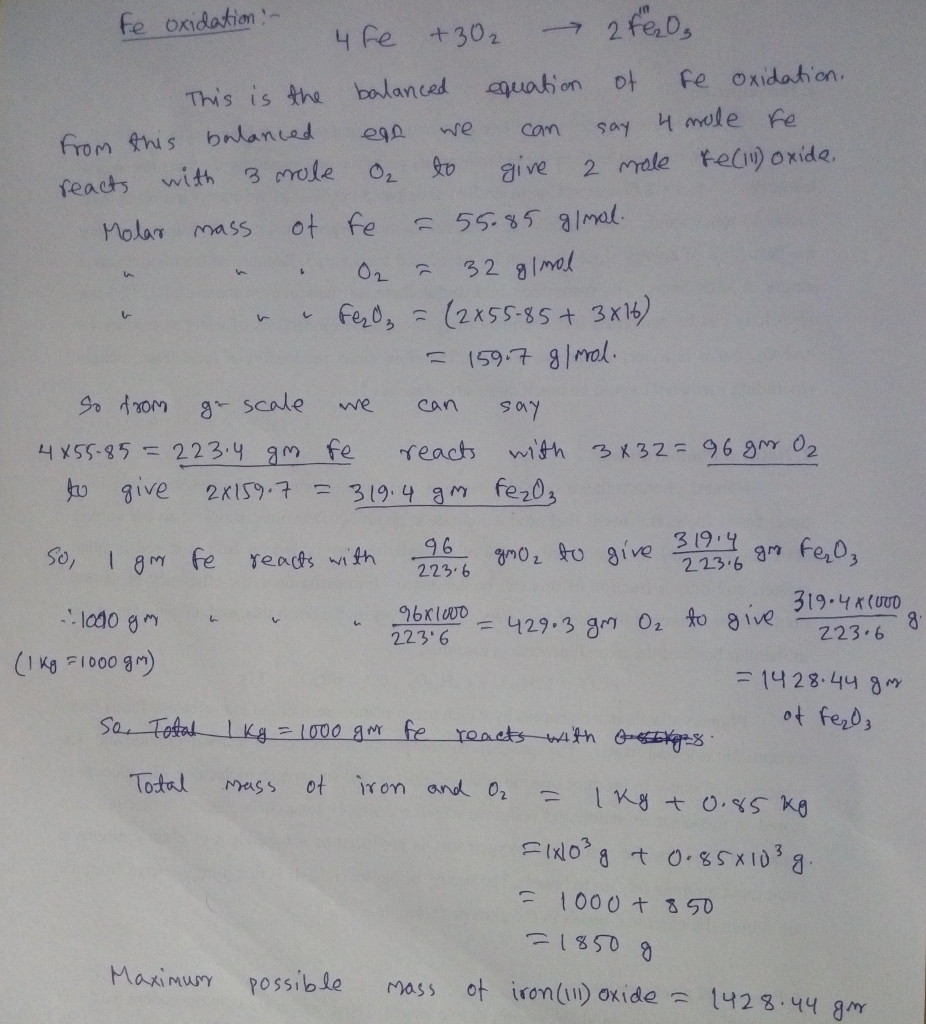In: Chemistry

# Model 2 - Oxidation of Iron C) 1.00 kg iron reacts with 0.86 kg oxygen from...

Model 2 - Oxidation of Iron C) 1.00 kg iron reacts with 0.86 kg oxygen from the atmosphere to produce iron(III) oxide {aka rust}.

In model C, what is the total mass of iron and oxygen in kg? Convert to your answer to grams and show how you would do this mathematical operation, and what is the maximum possible mass of iron(III) oxide product?

## Solutions

##### Expert Solution## Related Solutions

##### When the supply of oxygen is limited, iron metal reacts with oxygen to produce a mixture...
When the supply of oxygen is limited, iron metal reacts with oxygen to produce a mixture of FeO and Fe2O3 . In a certain experiment, 20.00 g iron metal was reacted with 11.31 g oxygen gas. After the experiment, the iron was totally consumed, and 3.91 g oxygen gas remained. Calculate the amounts of FeO and Fe2O3 formed in this experiment.
##### Solid Iron (II) Oxide reacts with Solid elemental iron and oxygen gas to create Iron (III)...
Solid Iron (II) Oxide reacts with Solid elemental iron and oxygen gas to create Iron (III) Oxide. Calculate ∆S​ * and determine the value for K of this reaction at 25C.
##### A 52 g sample of iron reacts with 22 g of oxygen to form how many...
A 52 g sample of iron reacts with 22 g of oxygen to form how many grams of iron oxide? Express your answer using two significant figures.
##### When 11.1 g of lead reacts with 3.81 L of oxygen gas, measured at 1.00 atm...
When 11.1 g of lead reacts with 3.81 L of oxygen gas, measured at 1.00 atm and 25.0 °C, 11.8 kJ of heat is released at constant pressure. What is ?H° for this reaction? (R = 0.0821 L • atm/(K • mol))2 Pb (s) + O2 (g) ? 2 PbO (s)
##### Calculate what is the mass in kg of 1.00-C of electrons
Calculate what is the mass in kg of 1.00-C of electrons
##### Five kg of oxygen (O​2​), initially at 430°C, fills a closed, rigid tank. Heat transfer from...
Five kg of oxygen (O​2​), initially at 430°C, fills a closed, rigid tank. Heat transfer from the oxygen occurs at the rate 425 W for 10 minutes. Assuming the ideal gas model with ​k ​ = 1.350 for the oxygen, determine its final temperature, in °C. Can't seem to find the right equations to use for this one, so I'm getting pretty stumped. Any help would be greatly appreciated
##### 6.103. A sample of oxygen was collected over water at 25°C and 1.00 atm. a. If...
6.103. A sample of oxygen was collected over water at 25°C and 1.00 atm. a. If the total sample volume was 0.480 L, how many moles of O2 were collected? b. If the same volume of oxygen is collected over ethanol instead of water, does it contain the same number of moles of O2? 6.104. Water and ethanol were removed from the O2 samples in Problem 6.103. a. What is the volume of the dry O2 gas sample at 25°C...
##### A 1.50-kg iron horseshoe initially at 550°C is dropped into a bucket containing 18.0 kg of...
A 1.50-kg iron horseshoe initially at 550°C is dropped into a bucket containing 18.0 kg of water at 20.0°C. What is the final temperature of the water–horseshoe system? Ignore the heat capacity of the container and assume a negligible amount of water boils away.
##### A 0.26 kg iron horseshoe that is initially at 787 ◦C is dropped into a bucket...
A 0.26 kg iron horseshoe that is initially at 787 ◦C is dropped into a bucket containing 20 kg of water at 34◦C. What is the final equilibrium temperature? Neglect any energy transfer to or from the surroundings and assume the specific heat of iron is 448 J/kg · ◦ C . The specific heat of water is 4186 J/kg · ◦ C . Answer in units of ◦C
##### An iron block of mass miron = 1 kg at a temperature of Tiron = 200°C...
An iron block of mass miron = 1 kg at a temperature of Tiron = 200°C is thrown in sub-cooled water of mass m at Twater = 25°C enclosed in an insulated container. Calculate and plot in Excel or in similar programs the entropy generated as a result of the heat transfer process for a range of water mass values between 10 kg and 10,000 kg. (Please submit your Excel sheet or your computer program with the homework.